Anzeige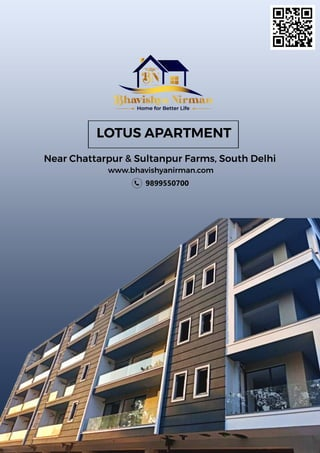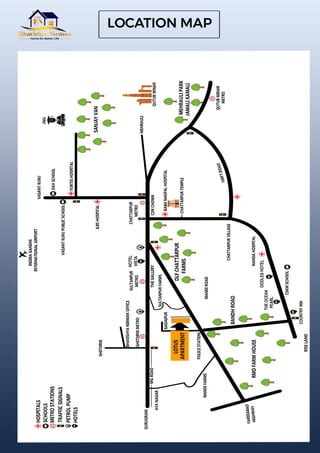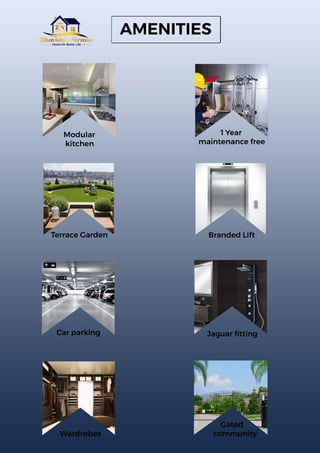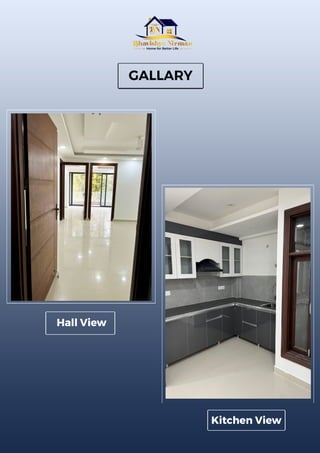Anzeige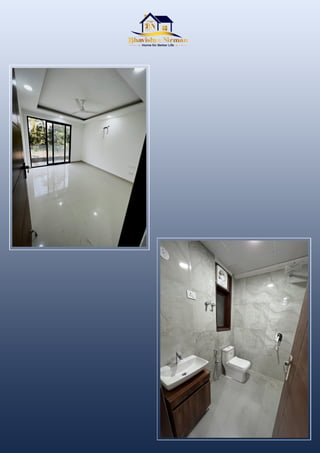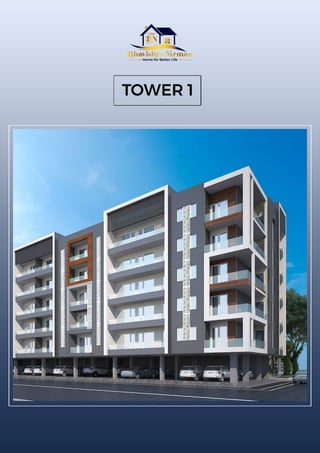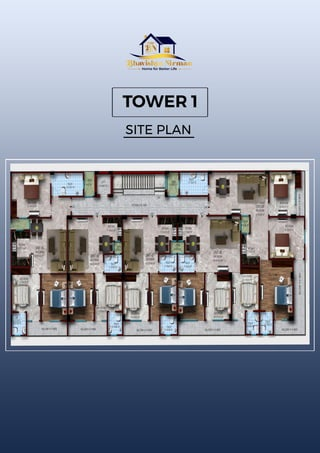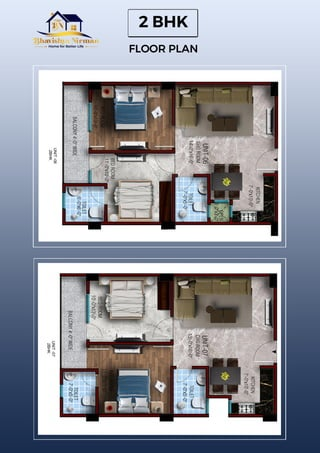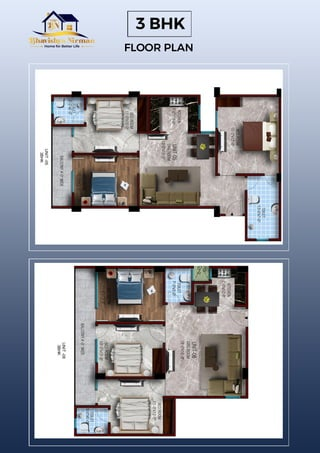Anzeige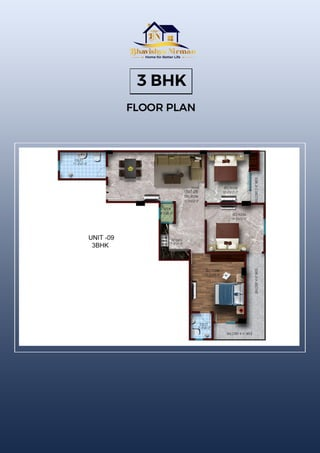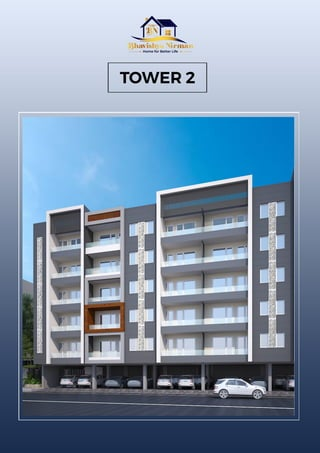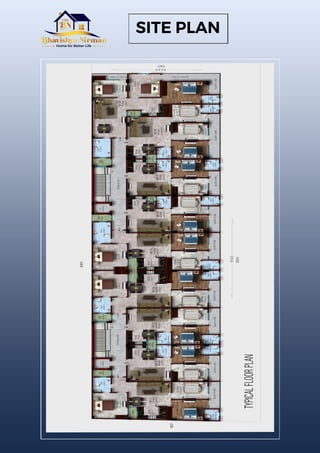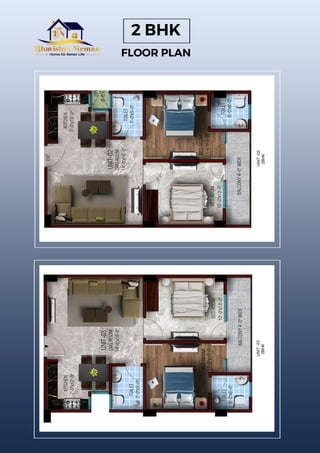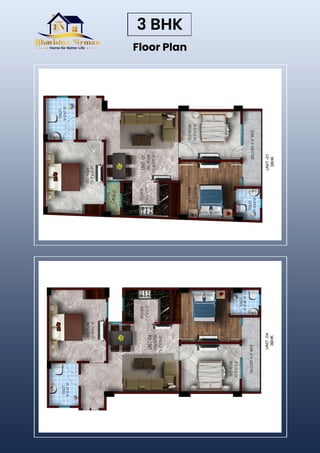Anzeige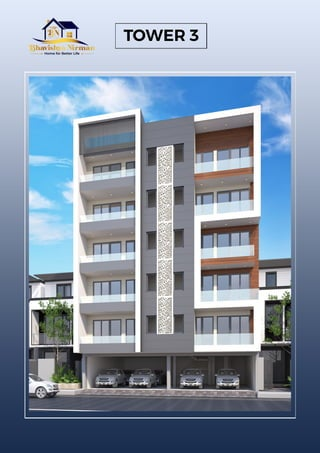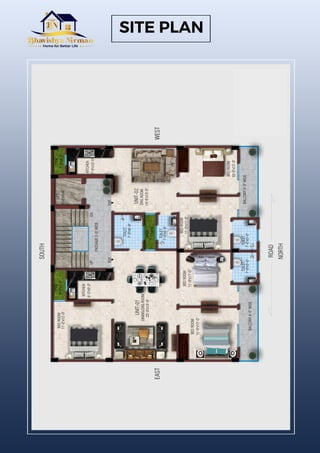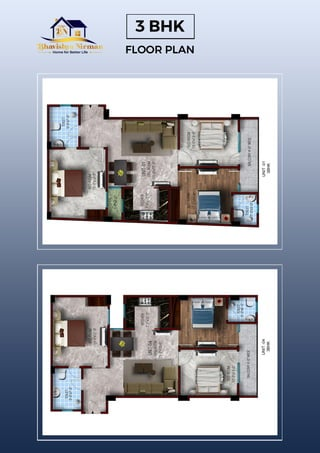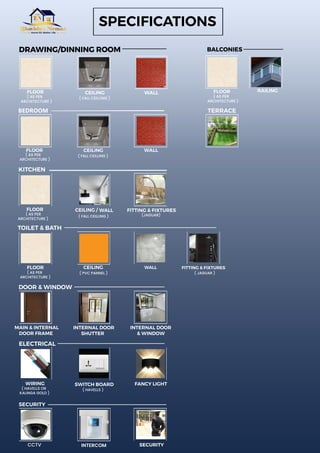Anzeige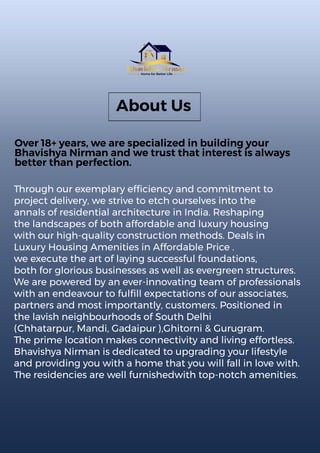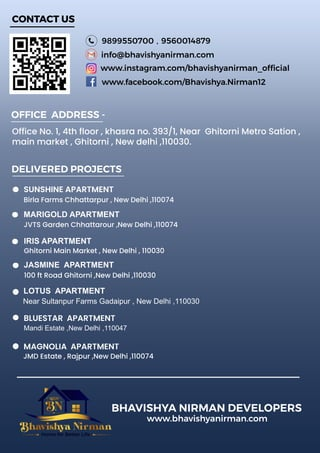Nächste SlideShare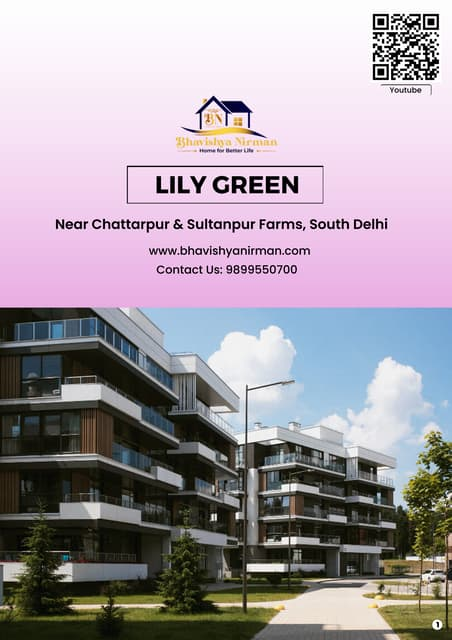2 BHK, 3 BHK & 4 BHK Ready to move Flats in Chattarpur, South Delhi |
1 von 21
Anzeige

### Flats in Chattarpur With Bank Loan

1. L O T U SA P A R T ME N T N e a r C h a t t a r p u r &S u l t a n p u r F a r ms , S o u t hD e l h i www. b h a v i s h y a n i r ma n . c o m 9 8 9 9 5 5 0 7 0 0
2. L O C A T I ONMA P
3. A ME N I T I E S Mo d u l a r k i t c h e n Wa r d r o b e s G a t e d c o mmu n i t y 1 Y e a r ma i n t e n a n c ef r e e T e r r a c eG a r d e n B r a n d e dL i f t C a r p a r k i n g J a g u a r ﬁ t t i n g
4. G A L L A R Y K i t c h e nV i e w H a l l V i e w
5. T O WE R1
6. T O WE R1 S I T EP L A N
7. 2B H K F L O ORP L A N
8. 3B H K F L O ORP L A N
9. 3B H K F L O ORP L A N
10. T O WE R2
11. S I T EP L A N
12. 2B H K F L O ORP L A N
13. 3B H K F l o o r P l a n
14. T O WE R3
15. S I T EP L A N
16. 3B H K F L O ORP L A N
17. S P E C I A L F E A T U R E S R E G I S T R Y L o a na v a i l a b l e T E R R A C EG A R D E N
18. S P E C I F I C A T I ON S C E I L I N G B A L C ON I E S F L O OR F L O OR R A I L I N G WA L L D R A WI N G / D I N N I N GR O OM ( A SP E R A R C H I T E C T U R E ) ( F A L L C E I L L I N G) ( A SP E R A R C H I T E C T U R E ) ( F A L L C E I L L I N G) C E I L I N G/ F L O OR WA L L F I T T I N G&F I X T U R E S K I T C H E N ( A SP E R A R C H I T E C T U R E ) ( J A G U A R ) C E I L I N G F L O OR WA L L F I T T I N G&F I X T U R E S T OI L E T&B A T H ( A SP E R A R C H I T E C T U R E ) ( P V CP A N N E L ) ( J A G U A R) ( F A L L C E I L L I N G) S E C U R I T Y C C T V I N T E R C O M S E C U R I T Y ( H A V E L L S) S WI T C HB O A R D E L E C T R I C A L ( H A V E L L SO R K A L I N G AG O L D) WI R I N G C E I L I N G F L O OR WA L L B E D R O O M T E R R A C E ( A SP E R A R C H I T E C T U R E ) F A N C YL I G H T I N T E R N A L D O OR &WI N D O W D O OR&WI N D O W I N T E R N A L D O OR S H U T T E R MA I N&I N T E R N A L D O ORF R A ME
19. A b o u t U s Ov e r 1 8 + y e a r s , wea r es p e c i a l i z e di nb u i l d i n gy o u r B h a v i s h y aN i r ma na n dwet r u s t t h a t i n t e r e s t i s a l wa y s b e t t e r t h a np e r f e c t i o n . T h r o u g ho u r e x e mp l a r y e f ﬁ c i e n c y a n dc o mmi t me n t t o p r o j e c t d e l i v e r y , wes t r i v et oe t c ho u r s e l v e s i n t ot h e a n n a l s o f r e s i d e n t i a l a r c h i t e c t u r ei nI n d i a . R e s h a p i n g t h el a n d s c a p e s o f b o t ha f f o r d a b l ea n dl u x u r y h o u s i n g wi t ho u r h i g h - q u a l i t y c o n s t r u c t i o nme t h o d s . D e a l s i n L u x u r y H o u s i n gA me n i t i e s i nA f f o r d a b l eP r i c e. w wee x e c u t et h ea r t o f l a y i n gs u c c e s s f u l f o u n d a t i o n s , b o t hf o r g l o r i o u s b u s i n e s s e s a s we l l a s e v e r g r e e ns t r u c t u r e s . Wea r ep o we r e db y a ne v e r - i n n o v a t i n gt e a m o f p r o f e s s i o n a l s wi t ha ne n d e a v o u r t of u l ﬁ l l e x p e c t a t i o n s o f o u r a s s o c i a t e s , p a r t n e r s a n dmo s t i mp o r t a n t l y , c u s t o me r s . P o s i t i o n e di n t h el a v i s hn e i g h b o u r h o o d s o f S o u t hD e l h i ( C h h a t a r p u r , Ma n d i , G a d a i p u r ) , G h i t o r n i &G u r u g r a m. T T h ep r i mel o c a t i o nma k e s c o n n e c t i v i t y a n dl i v i n ge f f o r t l e s s . B h a v i s h y aN i r ma ni s d e d i c a t e dt ou p g r a d i n gy o u r l i f e s t y l e a n dp r o v i d i n gy o uwi t hah o met h a t y o uwi l l f a l l i nl o v ewi t h . T h er e s i d e n c i e s a r ewe l l f u r n i s h e d wi t ht o p - n o t c ha me n i t i e s .
20. , MA G N O L I A A P A R T ME N T J MDE s t a t e, R a j p u r , N e wD e l h i , 1 1 0 0 7 4 B L U E S T A RA P A R T ME N T Ma n d i E s t a t e, N e wD e l h i , 1 1 0 0 4 7 L O T U S A P A R T ME N T N e a r S u l t a n p u r F a r ms G a d a i p u r , N e wD e l h i , 1 1 0 0 3 0 J A S MI N E A P A R T ME N T MA R I G O L DA P A R T ME N T J V T SG a r d e nC h h a t t a r o u r , N e wD e l h i , 1 1 0 0 7 4 S U N S H I N E A P A R T ME N T B i r l aF a r ms C h h a t t a r p u r , N e wD e l h i , 1 1 0 0 7 4 I R I SA P A R T ME N T G h i t o r n i Ma i nMa r k e t , N e wD e l h i , 1 1 0 0 3 0 1 0 0f t R o a dG h i t o r n i , N e wD e l h i , 1 1 0 0 3 0 OF F I C EA D D R E S S- O f f i c eN o . 1 , 4 t hf l o o r , k h a s r an o . 3 9 3 / 1 , N e a rG h i t o r n i Me t r oS a t i o n, ma i nma r k e t , G h i t o r n i , N e wd e l h i , 1 1 0 0 3 0 . D E L I V E R E DP R O J E C T S www. f a c e b o o k . c o m/ B h a v i s h y a . N i r ma n 1 2 www. i n s t a g r a m. c o m/ b h a v i s h y a n i r ma n _ o f ﬁ c i a l 9 5 6 0 0 1 4 8 7 9 i n f o @b h a v i s h y a n i r ma n . c o m 9 8 9 9 5 5 0 7 0 0 C ON T A C TU S www. b h a v i s h y a n i r ma n . c o m B H A V I S H Y AN I R MA ND E V E L OP E R S
Anzeige